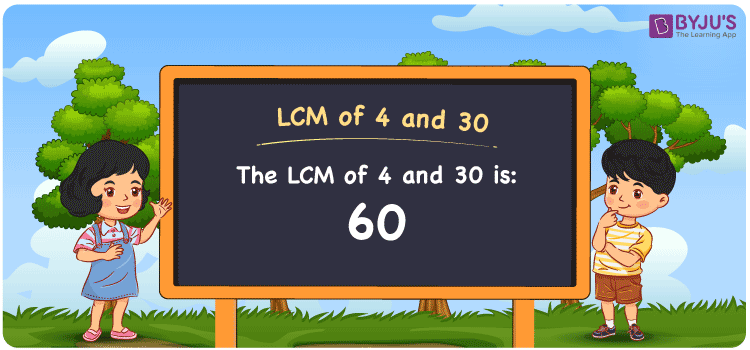# LCM of 4 and 30

LCM of 4 and 30 is 60. The LCM of any two integers in math is the value that is evenly divisible by the two values. Among all common multiples of 4 and 30, the LCM of 4 and 30 is the smallest value. (4, 8, 12, 16, 20, 24, 28,…) and (30, 60, 90, 120, 150, 180,…) are the first few multiples of four and thirty, respectively. To find the LCM of 4 and 30, you can use one of three methods: listing multiples, prime factorization, or division.

Also read: Least common multiple

## What is LCM of 4 and 30?

The answer to this question is 60. The LCM of 4 and 30 using various methods is shown in this article for your reference. The LCM of two non-zero integers, 4 and 30, is the smallest positive integer 60 which is divisible by both 4 and 30 with no remainder.## How to Find LCM of 4 and 30?

LCM of 4 and 30 can be found using three methods:

• Prime Factorisation
• Division method
• Listing the multiples

### LCM of 4 and 30 Using Prime Factorisation Method

The prime factorisation of 4 and 30, respectively, is given by:

4 = (2 × 2) = 22 and

30 = (2 × 3 × 5) = 21 × 31 × 51

LCM (4, 30) = 60

### LCM of 4 and 30 Using Division Method

We’ll divide the numbers (4, 30) by their prime factors to get the LCM of 4 and 30 using the division method (preferably common). The LCM of 4 and 30 is calculated by multiplying these divisors.

 2 4 30 2 2 15 3 1 15 5 1 5 x 1 1

No further division can be done.

Hence, LCM (4, 30) = 60

### LCM of 4 and 30 Using Listing the Multiples

To calculate the LCM of 4 and 30 by listing out the common multiples, list the multiples as shown below

 Multiples of 4 Multiples of 30 4 30 8 60 12 90 ….. 120 60 150

The smallest common multiple of 4 and 30 is 60.

Therefore LCM (4, 30) = 60

## Video Lesson on Applications of LCM## LCM of 4 and 30 Solved Example

The product of two numbers is 120. If their GCD is 2, what is their LCM?

Solution:

Given: GCD = 2

product of numbers = 120

∵ LCM × GCD = product of numbers

⇒ LCM = Product/GCD = 120/2

Therefore, the LCM is 60.

The probable combination for the given case is LCM(4, 30) = 60.

## Frequently Asked Questions on LCM of 4 and 30

### What is the LCM of 4 and 30?

The LCM of 4 and 30 is 60. To find the LCM (least common multiple) of 4 and 30, we need to find the multiples of 4 and 30 (multiples of 4 = 4, 8, 12, 16 . . . . 60; multiples of 30 = 30, 60, 90, 120) and choose the smallest multiple that is exactly divisible by 4 and 30, i.e., 60.

### List the methods used to find the LCM of 4 and 30.

The methods used to find the LCM of 4 and 30 are Prime Factorization Method, Division Method and Listing multiples.

### What is the Relation Between GCF and LCM of 4, 30?

The following equation can be used to express the relation between GCF and LCM of 4 and 30, i.e. GCF × LCM = 4 × 30.

### If the LCM of 30 and 4 is 60, Find its GCF.

LCM(30, 4) × GCF(30, 4) = 30 × 4
Since the LCM of 30 and 4 = 60
⇒ 60 × GCF(30, 4) = 120
Therefore, the GCF = 120/60 = 2.

### What is the Least Perfect Square Divisible by 4 and 30?

The least number divisible by 4 and 30 = LCM(4, 30)
LCM of 4 and 30 = 2 × 2 × 3 × 5 [Incomplete pair(s): 3, 5] ⇒ Least perfect square divisible by each 4 and 30 = LCM(4, 30) × 3 × 5 = 900 [Square root of 900 = √900 = ±30] Therefore, 900 is the required number.